XbarR HOWTO

2018-11-30

XbarR charts are useful when monitoring a continuous process over time. Some examples might include (i) parts coming off an assembly line, (ii) molded parts produced several at a time over several cycles, or (iii) batch uniformity of continuously produced chemical.

Generating an Xbar or XbarS plot with ggQC is simple. To get us started, let’s simulate some production line data on candles. The candles are shaped using a mold capable of producing 4 units a cycle. Each cycle takes an hour. Thus in a 24 hour period, the process would yield 96 candles. The parameter being tracked is candle width.

set.seed(5555)
candle_df1t3 <- data.frame(
Cycle = as.factor(rep(1:24, each=3)),
candle_width = rnorm(n = 3*24, mean = 10, sd = 1),
mold_cell = as.ordered(rep(1:3))
)

candle_df4 <- data.frame(
Cycle = as.factor(rep(1:24, each=1)),
candle_width = rnorm(n = 1*24, mean = 11, sd = 2),
mold_cell = as.ordered(rep(4, each=24))
)

candle_df <- rbind(candle_df1t3, candle_df4)
library(ggplot2)
library(ggQC)

Simple XbarR Plot

Making a plot with ggQC and ggplot is simple

NOTEs:

• Remember to set the group aesthetic equal to 1. Otherwise you will end up with far more control lines than you want.
• XbarR is the default method for stat_QC and stat_QC_labels functions.
XbarR <- ggplot(candle_df, aes(x = Cycle, y = candle_width, group = 1)) +
stat_summary(fun.y = mean, geom = "point") +
stat_summary(fun.y = mean, geom = "line") +
stat_QC()

XbarR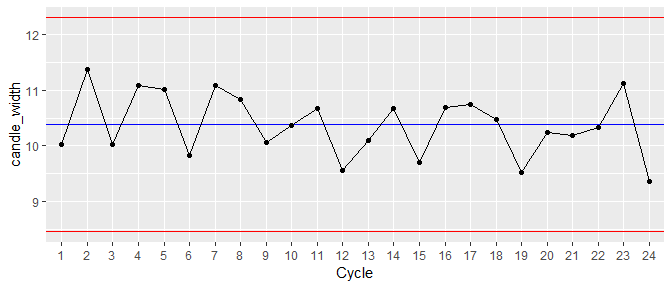Data looks to be in good control, but it would be nice the have the center line and control limits labeled before presenting it to the line manager.

Labeled XbarR Plot

XbarR + stat_QC_labels()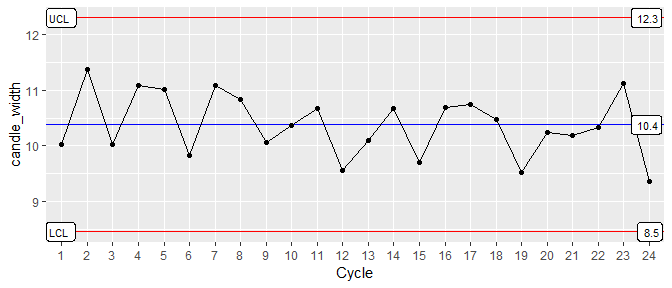Your line manager is happy to see the candles are being produced as intended, but would like to get a sense of the process consistency. For this you will need an R-Bar chart.

R Bar Chart

R_Bar <- ggplot(candle_df, aes(x = Cycle, y = candle_width, group = 1)) +
stat_summary(fun.y = QCrange, geom = "point") +
stat_summary(fun.y = QCrange, geom = "line") +
stat_QC(method="rBar") +
stat_QC_labels(method="rBar") + ylab("R-Bar")

R_Bar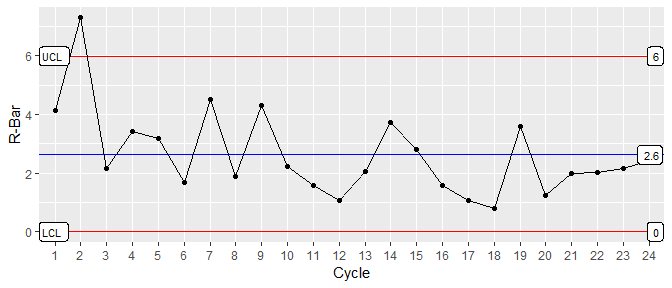The second run for the day was more inconsistent than usual, and the line manager lifts an eye brow. He has just received a customer complaint that some of the candles are too wide to fit it their candle holders. He asks if you can show the individuals on the plot and the natural control limits.

XbarR with Individuals

XbarR <- ggplot(candle_df, aes(x = Cycle, y = candle_width, group = 1)) +
stat_summary(fun.y = mean, geom = "point") +
stat_summary(fun.y = mean, geom = "line") +
stat_QC() + stat_QC_labels() +
# Show Individuals
geom_point(alpha= 1/5) +
stat_QC(n=1, color.qc_limits = "orange") +
stat_QC_labels(n=1, color.qc_limits = "orange")

XbarR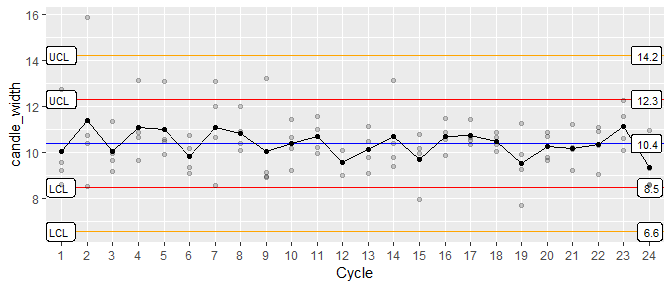The line manager is surprised to see so many widths over 13 units and wants you to examine the data as a function of the different cells in the mold

Colorizing the Data

XbarR <- ggplot(candle_df, aes(x = Cycle, y = candle_width, group = 1, color=mold_cell)) +
stat_summary(fun.y = mean, geom = "point") +
stat_summary(fun.y = mean, geom = "line") +
stat_QC() + stat_QC_labels() +
# Show Individuals
geom_point(alpha= 1/2) +
stat_QC(n=1, color.qc_limits = "orange") +
stat_QC_labels(n=1, color.qc_limits = "orange")

XbarR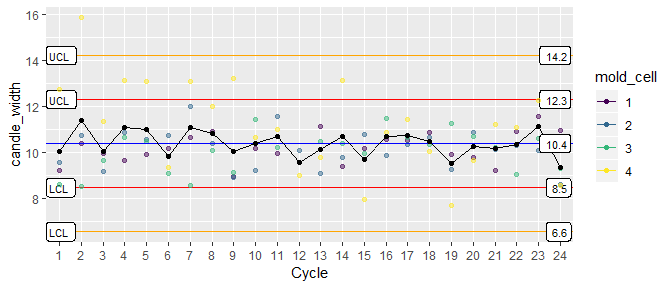Mold Cell 4 looks a little suspicious. So you plot an XmR chart for each cell.

Faceting

XmR <- ggplot(candle_df,
aes(x = Cycle, y = candle_width, group = 1, color = mold_cell)) +
geom_point() + geom_line() +
stat_QC(method="XmR") + stat_QC_labels(method="XmR") +
facet_grid(.~mold_cell)

XmR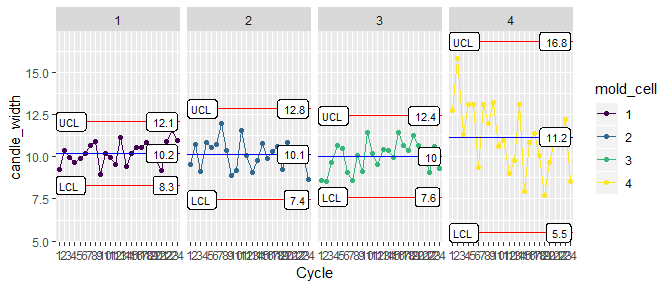Nice work! Looks like we need to replace the mold.

For more information and examples using ggQC please visit (ggQC.r-bar.net)